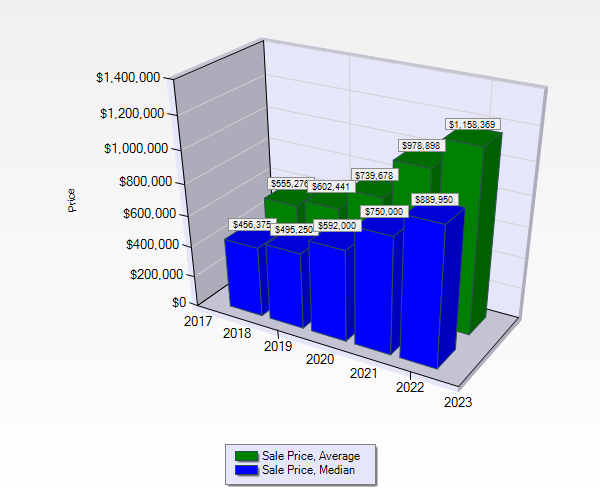July 26th, 2023

# Which Is Better Average or Median Home Price?### Which is Better? – Average Bozeman Home Price or Median Bozeman Home Price? – Which is Best?

Average and median home prices in Bozeman can be significantly different, so it’s important to compare average price to median price and to understand the differences between these 2, easily mastered, formulas.

#### —— Average Home Price ——

When looking at Bozeman home price statistics, average price is often inaccurate because the area has a small population in comparison to, say, Billings or Boise. But this formula can be better in many cases.

Average price, aka, mean, is found by simply adding all prices, e.g., \$50,000; \$90,000, \$150,000, \$330,000, \$470,000 = \$1,090,000 and then dividing the sum, \$1,090,000, of these numbers by the quantity–5–of these numbers.

Thus, \$1,090,000 divided by 5 = \$218,000, which is the average price.

Average price often depicts wild price swings, throwing off your search for a reasonable reflection of Bozeman home prices.

Average is better* when prices are relatively close in value, e.g. \$150,000, 158,000, 165,000, etc. But as explained above, it’s not as accurate a reflection of home prices when prices are outliers, i.e., far apart.

#### —— Median Home Price ——

Used as an numerical phrase, median signifies a middle price in a set of Bozeman house prices.

Frankly, using the median price results in a better depiction of the typical or the middle price than using average price.

To find median price, line up the prices, in this case 5 prices, an odd number, in order of prices, e.g., \$50,000; \$90,000, \$150,000, \$330,000, \$470,000, and simply pick the middle price, so median is simply \$150,000.

Now, if the quantity of prices is even–4 in this case–\$50,000; \$150,000, \$330,000, and \$470,000, there is no “middle” price to take as your mean.

Therefore, you start the calculation by taking the average of the 2 middle prices:

\$150,000 + \$330,000 = \$480,000, and then divide \$480,000 by 2 = \$240,000.

Next, you add \$240,000 to both \$50,000 and \$470,000.

So, \$240,000 + \$50,000 + \$470,000 = \$760,000, divided by 3 = \$253,333

Therefore, you would use this number, \$253,333, as your median price.

#### —— Conclusion ——

In conclusion, the median price formula is usually the more accurate of the 2, so, for the most part, median is best.

But, as mentioned above*, the average price formula has its place and can be better, depending on your statistical need.

Both numbers have their benefit in studying Bozeman home prices, so don’t rule either one out!

Note: If you are using fewer than 30 home prices in either formula, your final home price–average or median–may not be accurate enough to reflect your real estate market’s home prices, so look at other factors, too.